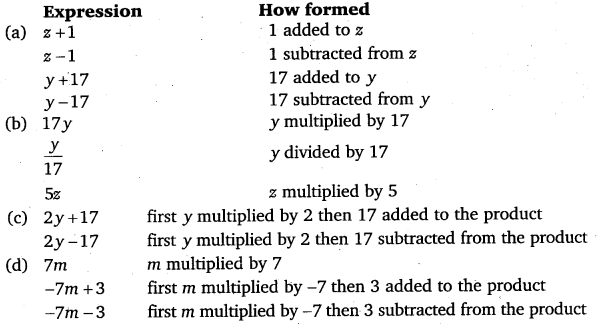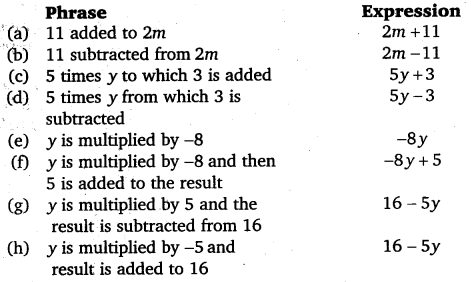# Class 6 Maths NCERT Solutions for Chapter 11 Algebra Ex – 11.3

## Algebra

Question 1.
Make up as many expressions with numbers (no variables) as you can from three numbers 5, 7 and 8. Every number should be used not more than once. Use only addition, subtraction and multiplication.

Solution:
Some possible expressions using three numbers 5, 7 and 8 are
(i) 5 + (8 – 7)
(ii) 5 -(8 – 7)
(iii) 5 +(8 + 7)
(iv) (5 + 8)+ 7
(v) 5 x 8 + 7
(vi) 5 x 7 + 8
(vii) 5 x 8 – 7
(viii) 5 x 7 – 8
(ix) 5 x (8 – 7)
(x) 5 x (8 + 7)
(xi) 8 x (7 – 5)
(xii) 8 x (7 + 5) etc.

Question 2.
Which out of the following are expressions with numbers only?

(a) y + 3
(b) (7 x 20) – 8z
(c) 5(21 – 7) + 7 x 2
(d) 5
(e) 3x
(f) 5 – 5n
(g) (7 x 20) (5 x 10) – 45 + p

Solution:
(c) and (d) are expressions with numbers only.

Question 3.
Identify the operations (addition, subtraction, division, multiplication) in forming the following expressions and tell how the expressions have been formed:

(a) z +1, z – 1 y +17, y -17
(b) 17y,$\frac { y }{ 17 }$, 5z
(c) 2y +17, 2y -17
(d) 7m, -7m + 3, -7m – 3

Solution:Question 4.
Give expressions for the following cases:

(b) 7 subtracted from p
(c) p multiplied by 7
(d) p divided by 7
(e) 7 subtracted from -m
(f) -p multiplied by 5
(g) -p divided by 5
(h) p multiplied by -5

Solution:Question 5.
Give expressions in the following cases:

(b) 11 subtracted from 2m
(c) 5 times y to which 3 is added
(d) 5 times y from which 3 is subtracted
(e) y is multiplied by -8
(f) y is multiplied by -8 and then 5 is added to the result
(g) y is multiplied by 5 and the result is subtracted from 16
(h) y is multiplied by -5 and the result is added to 16

Solution:Question 6.
(a) Form expressions using t and 4. Use not more than one number operation. Every expression must have t in it.
(b) Form expressions using y, 2 and 7. Every expression must have y in it. Use only two number operations. These should be different.

Solution:
(a) Possible expressions using t and 4 are
t + 4, t – 4, 4t and$\frac { t }{ 4 }$.
(b) Possible expressions using y, 2 and 7 (having only two different number operations) are
2y + 7, 2y – 7, 7y + 2, 7y – 2,$\frac { y }{ 2 }$ + 7,$\frac { y }{ 2 }$ – 7,$\frac { y }{ 7 }$+2 and$\frac { y }{ 7 }$ – 2.